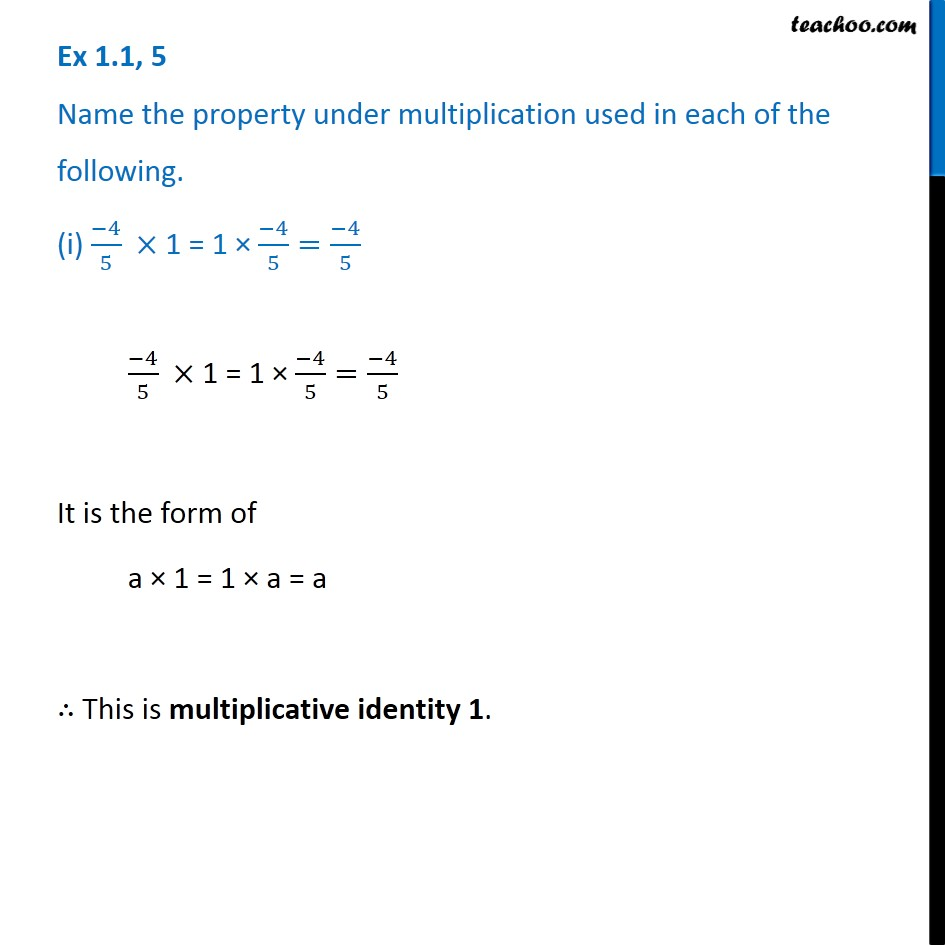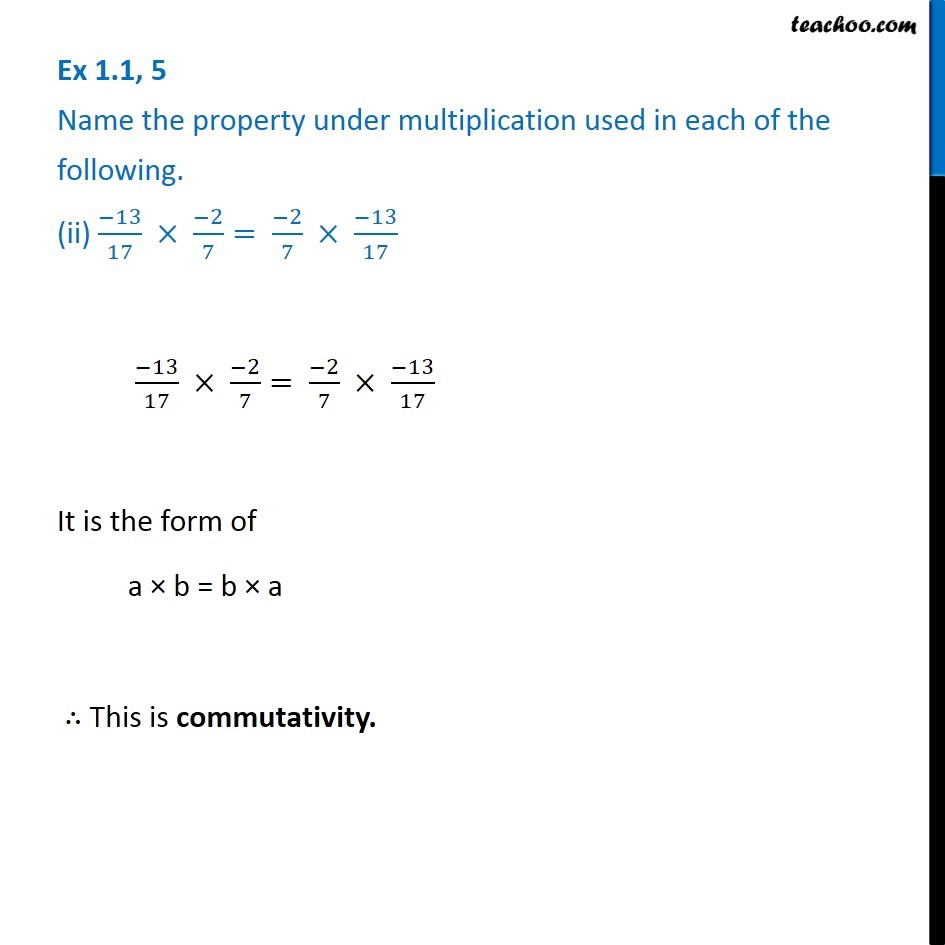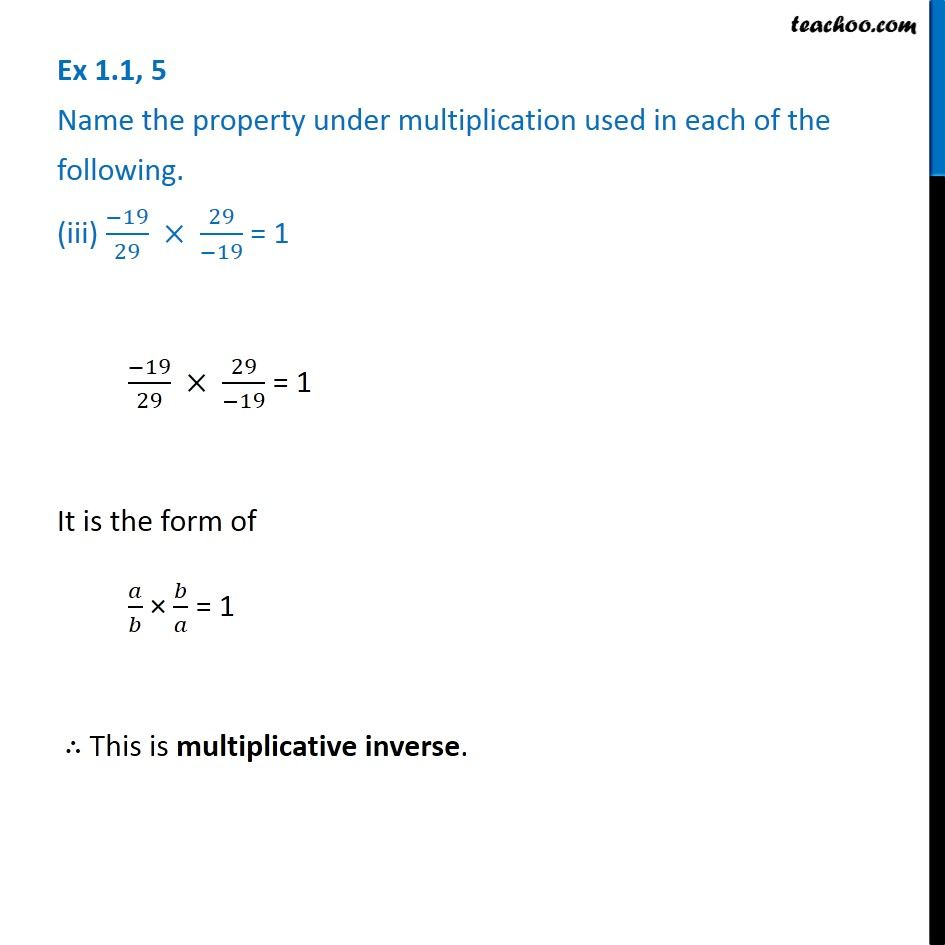Ex 1.1

Chapter 1 Class 8 Rational Numbers
Serial order wiseGet live Maths 1-on-1 Classs - Class 6 to 12

### Transcript

Ex 1.1, 5 Name the property under multiplication used in each of the following. (i) (−4)/5 × 1 = 1 × (−4)/5=(−4)/5 (−4)/5 × 1 = 1 × (−4)/5=(−4)/5 It is the form of a × 1 = 1 × a = a ∴ This is multiplicative identity 1. Ex 1.1, 5 Name the property under multiplication used in each of the following. (ii) (−13)/17 × (−2)/7= (−2)/7 × (−13)/17 (−13)/17 × (−2)/7= (−2)/7 × (−13)/17 It is the form of a × b = b × a ∴ This is commutativity. Ex 1.1, 5 Name the property under multiplication used in each of the following. (iii) (−19)/29 × 29/(−19) = 1 (−19)/29 × 29/(−19) = 1 It is the form of 𝑎/𝑏 × 𝑏/𝑎 = 1 ∴ This is multiplicative inverse.